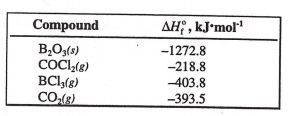# Problem: Use the given heats of formation to calculate the enthalpy change for this reaction. B2O3 (s) + 3 COCl2 (g) → 2 BCl3 (g) + 3 CO2 (g) a) 694.3 kJ b) 354.9 kJ c) -58.9 kJ d) -3917.3 kJ

###### FREE Expert Solution
83% (213 ratings)
###### Problem Details

Use the given heats of formation to calculate the enthalpy change for this reaction.

B2O(s) + 3 COCl(g) → 2 BCl(g) + 3 CO(g)

a) 694.3 kJ

b) 354.9 kJ

c) -58.9 kJ

d) -3917.3 kJWhat scientific concept do you need to know in order to solve this problem?

Our tutors have indicated that to solve this problem you will need to apply the Enthalpy of Formation concept. You can view video lessons to learn Enthalpy of Formation. Or if you need more Enthalpy of Formation practice, you can also practice Enthalpy of Formation practice problems.

What is the difficulty of this problem?

Our tutors rated the difficulty ofUse the given heats of formation to calculate the enthalpy c...as medium difficulty.

How long does this problem take to solve?

Our expert Chemistry tutor, Sabrina took 4 minutes and 44 seconds to solve this problem. You can follow their steps in the video explanation above.

What professor is this problem relevant for?

Based on our data, we think this problem is relevant for Professor Graves' class at FIU.